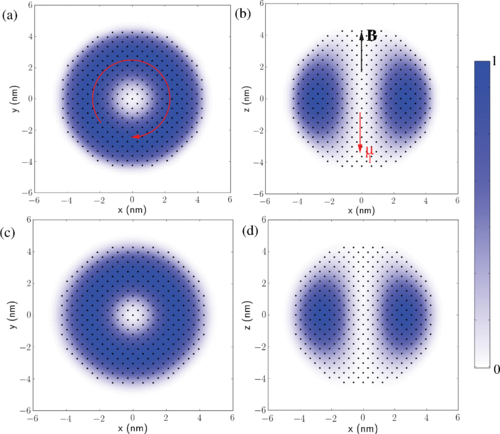# Séminaire autour de la manipulation de spin dans les nanostructures de semiconducteurs

#### Physics of electron g-factors in semiconductor nanostructures

Athmane Tadjine (doctorant dans le groupe physique)
Salle du conseil, mardi 16 novembre 2017, 14h00.Normalized density of g0−gz on each atom of a spherical nanocrystal of CdSe (diameter = 9 nm) for a magnetic field along z. The density is shown in the xOy (a) and xOz (b) planes passing through the center of the sphere. These data can be seen as the intensity of the local orbital component μl(r) of the magnetic moment (red arrow) induced by the circulating current [depicted by the circular arrow in (a)] generated by the spin-orbit coupling. The atoms are represented by black dots. (c) and (d) are same as (a) and (b), respectively, but calculated using the analytic envelope wave function

The manipulation of the electron spin in semiconductor nanostructures requires the knowledge of the electron g-factor. In this work, we revisit the physics of the electron g-factor in nanostructures of various shape, size, dimensionality (0D-3D) and composition. Our investigation is based on a combination of atomistic and analytical calculations.

We show that, for a given compound, the electron g-factors follow a universal law that just depends on the energy gap, in particular along rotational symmetry axes. We demonstrate that the orbital magnetic moment density strongly depends on the shape of the nanostructure but the total (integrated) magnetic moment is independent of the shape and therefore of the electron envelope wavefunction. The physical origin of this non-trivial behavior is explained.
We deduce that the bulk component of the g-factor is isotropic and that g-factor anisotropies entirely come from surface effects.

Athmane Tadjine (1), Yann-Michel Niquet (2), and Christophe Delerue (1)

1 Univ. Lille, CNRS, Centrale Lille, ISEN, Univ. Valenciennes, UMR 8520-IEMN,F-59000 Lille, nFrance
2 Université Grenoble Alpes, INAC-MEM, L Sim, Grenoble, France and CEA, INAC-MEM, L Sim, 38000 Grenoble, France

Reference: A. Tadjine, Y.-M. Niquet, and C. Delerue, Phys. Rev. B 95, 235437 (2017).

# Séminaire autour de la manipulation de spin dans les nanostructures de semiconducteurs

#### Physics of electron g-factors in semiconductor nanostructures

Athmane Tadjine (doctorant dans le groupe physique)
Salle du conseil, mardi 16 novembre 2017, 14h00.Normalized density of g0−gz on each atom of a spherical nanocrystal of CdSe (diameter = 9 nm) for a magnetic field along z. The density is shown in the xOy (a) and xOz (b) planes passing through the center of the sphere. These data can be seen as the intensity of the local orbital component μl(r) of the magnetic moment (red arrow) induced by the circulating current [depicted by the circular arrow in (a)] generated by the spin-orbit coupling. The atoms are represented by black dots. (c) and (d) are same as (a) and (b), respectively, but calculated using the analytic envelope wave function

The manipulation of the electron spin in semiconductor nanostructures requires the knowledge of the electron g-factor. In this work, we revisit the physics of the electron g-factor in nanostructures of various shape, size, dimensionality (0D-3D) and composition. Our investigation is based on a combination of atomistic and analytical calculations.

We show that, for a given compound, the electron g-factors follow a universal law that just depends on the energy gap, in particular along rotational symmetry axes. We demonstrate that the orbital magnetic moment density strongly depends on the shape of the nanostructure but the total (integrated) magnetic moment is independent of the shape and therefore of the electron envelope wavefunction. The physical origin of this non-trivial behavior is explained.
We deduce that the bulk component of the g-factor is isotropic and that g-factor anisotropies entirely come from surface effects.

Athmane Tadjine (1), Yann-Michel Niquet (2), and Christophe Delerue (1)

1 Univ. Lille, CNRS, Centrale Lille, ISEN, Univ. Valenciennes, UMR 8520-IEMN,F-59000 Lille, nFrance
2 Université Grenoble Alpes, INAC-MEM, L Sim, Grenoble, France and CEA, INAC-MEM, L Sim, 38000 Grenoble, France

Reference: A. Tadjine, Y.-M. Niquet, and C. Delerue, Phys. Rev. B 95, 235437 (2017).